# 2023年成考高起点每日一练《数学(理)》11月21日

## 试卷预览

• 1. 已知空间向量i,j,k为两两垂直的单位向量，向量a=2i+3j+mk，若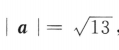，则m=（）

A-2

B-1

C0

D1

• 2. 某类灯泡使用时数在1000小时以上的概率为0.2,三个灯泡在使用1000小时以后最多只有一个坏的概率为（）

A0.008

B0.104

C0.096

D1

• 3. 已知集合M =(2,3,5,a),N =(1,3,4,b),若M∩N=(1,2,3),则a,b的值为

Aa=2,b=1

Ba=1,b=1

Ca=1,b= 2

Da=1,b=5

• 4. 中心在坐标原点，对称轴为坐标轴，且一个顶点（3，0），虚轴长为8的双曲线方程是（）

A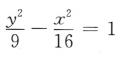B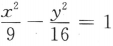C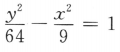D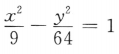• 1. 在△ABC中，B=120°,BC=4,△ABC的面积为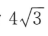，求AC.
• 2. 设函数f(x)=xlnx+x.(I）求曲线y=f(x）在点（(1,f(1))处的切线方程；
(II）求f（x）的极值．
• 3. 建筑一个容积为8000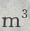,深为6m的长方体蓄水池，池壁每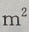的造价为15元，池底每的造价为30元。（I）把总造价y(元)表示为长x(m)的函数；（Ⅱ）求函数的定义域
• 4. 设函数f(x)=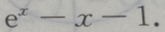(Ⅰ)求f(x)的单调区间; (Ⅱ)求 f(x)的极值
• 1. 长方体的长、宽、高分别为2,3,6，则该长方体的对角线长为（）
• 2. 若平面向量a=(x,1),b=(1,-2),且a//b,则x=（）